Next: Wave Packets Up: Wave Mechanics Previous: Schrödinger's Equation

# Probability Interpretation of Wavefunction

After many false starts, physicists in the early 20th century eventually came to the conclusion that the only physical interpretation of a particle wavefunction that is consistent with experimental observations is probabilistic in nature. To be more exact, if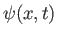is the complex wavefunction of a given particle, moving in one dimension along the-axis, then the probability of finding the particle betweenand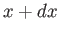at time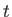is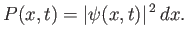(C.30)

We can interpret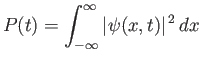(C.31)

as the probability of the particle being found anywhere between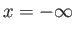and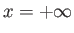at time. This follows, via induction, from the fundamental result in probability theory that the probability of the occurrence of one or other of two mutually exclusive events (such as the particle being found in two non-overlapping regions) is the sum (or integral) of the probabilities of the individual events. (See Section 2.3.) Assuming that the particle exists, it is certain that it will be found somewhere betweenandat time. Because a certain event has probability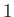(see Section 2.2), our probability interpretation of the wavefunction is only tenable provided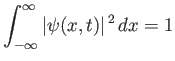(C.32)

at all times. A wavefunction that satisfies the previous condition--which is known as the normalization condition--is said to be properly normalized.

Suppose that we have a wavefunction,, which is such that it satisfies the normalization condition (C.32) at time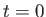. Furthermore, let the wavefunction evolve in time according to Schrödinger's equation, (C.24). Our probability interpretation of the wavefunction only makes sense if the normalization condition remains satisfied at all subsequent times. This follows because if the particle is certain to be found somewhere on the-axis (which is the interpretation put on the normalization condition) at timethen it is equally certain to be found somewhere on the-axis at a later time (because we are not considering any physical process by which particles can be created or destroyed). Thus, it is necessary for us to demonstrate that Schrödinger's equation preserves the normalization of the wavefunction.

Taking Schrödinger's equation, and multiplying it by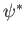(the complex conjugate of the wavefunction), we obtain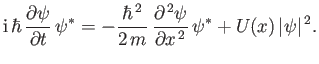(C.33)

The complex conjugate of the previous expression yields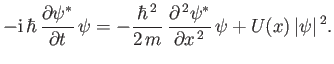(C.34)

Here, use has been made of the readily demonstrated results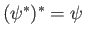and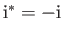, as well as the fact that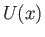is real. Taking the difference between the previous two expressions, we obtain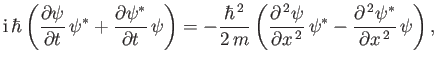(C.35)

which can be written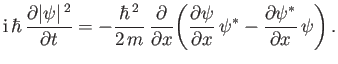(C.36)

Integrating in, we get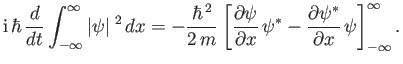(C.37)

Finally, assuming that the wavefunction is localized in space: that is,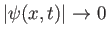as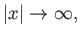(C.38)

we obtain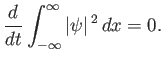(C.39)

It follows, from the preceding analysis, that if a localized wavefunction is properly normalized at(i.e., if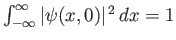) then it will remain properly normalized as it evolves in time according to Schrödinger's equation. Incidentally, a wavefunction that is not localized cannot be properly normalized, because its normalization integral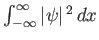is necessarily infinite. For such a wavefunction,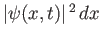gives the relative, rather than the absolute, probability of finding the particle betweenandat time. In other words, [cf., Equation (C.30)]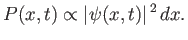(C.40)Next: Wave Packets Up: Wave Mechanics Previous: Schrödinger's Equation
Richard Fitzpatrick 2016-01-25